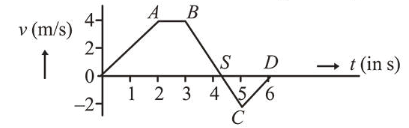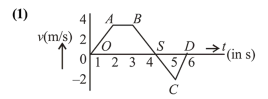# The velocity v and time t graph of a body in a straight line motion is shown in the figureQuestion:

The velocity $(v)$ and time $(t)$ graph of a body in a straight line motion is shown in the figure. The point $S$ is at $4.333$ seconds. The total distance covered by the body in $6 \mathrm{~s}$ is:1. (1) $\frac{37}{3} \mathrm{~m}$

2. (2) $12 \mathrm{~m}$

3. (3) $11 \mathrm{~m}$

4. (4) $\frac{49}{4} \mathrm{~m}$

Correct Option: 1

Solution:$O S=4+\frac{1}{3}=\frac{13}{3}$

$S D=2-\frac{1}{3}=\frac{5}{3}$

Distance covered by the body $=$ area of $v-t$ graph

$=\operatorname{ar}(O A B S)+\operatorname{ar}(S C D)$

$=\frac{1}{2}\left(\frac{13}{3}+1\right) \times 4+\frac{1}{2} \times \frac{5}{3} \times 2$

$=\frac{32}{3}+\frac{5}{3}=\frac{37}{3} \mathrm{~m}$